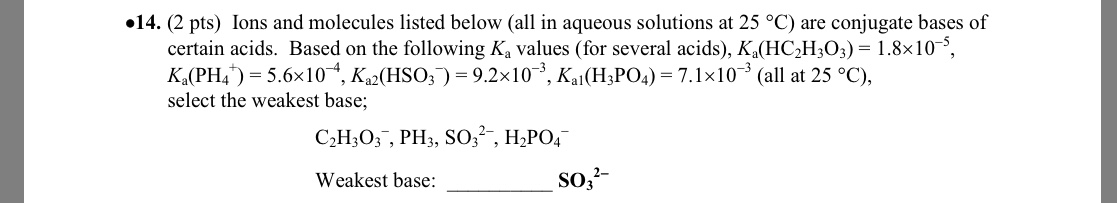# Ions and molecules listed below (all in aqueous solutions at 25 oC) are conjugate bases of certain acids. Based on the following Ka values (for several acids), Ka(HC2H3O2) = 1.8x10^-5, Ka(PH4^+) = 5.6x10^-4, Ka2(HSO3^-) = 9.2x10^-3, Ka1(H3PO4) = 7.1x10^-3 (all at 25 oC), select the weakest base.